# A.5 Spatial velocities and forces

Given two 3D coordinate frames A and B, the spatial velocity, or twist,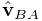of B with respect to A is given by the 6D composition of the translational velocity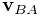of the origin of B with respect to A and the angular velocity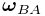: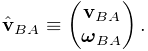(A.24)

Similarly, the spatial force, or wrench,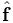acting on a frame B is given by the 6D composition of the translational force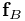acting on the frame’s origin and the moment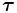, or torque, acting through the frame’s origin: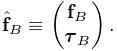(A.25)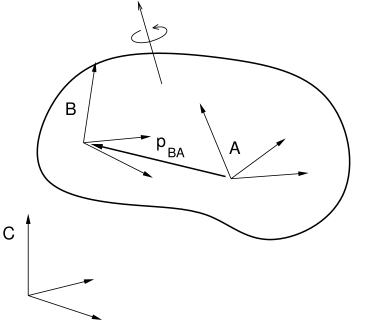Figure A.9: Two frames A and B rigidly connected within a rigid body and moving with respect to a third frame C.

If we have two frames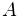and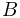rigidly connected within a rigid body (Figure A.9), and we know the spatial velocity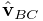ofwith respect to some third frame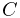, we may wish to know the spatial velocity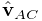ofwith respect to. The angular velocity components are the same, but the translational velocity components are coupled by the angular velocity and the offset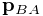betweenand, so that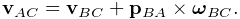is hence related tovia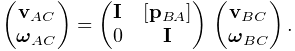where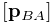is defined by (A.22).

The above equation assumes that all quantities are expressed with respect to the same coordinate frame. If we instead considerandto be represented in framesand, respectively, then we can show that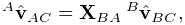(A.26)

where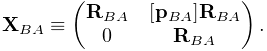(A.27)

The transform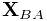is easily formed from the components of the rigid transform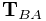relatingto.

The spatial forces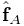and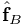acting on framesandwithin a rigid body are related in a similar way, only with spatial forces, it is the moment that is coupled through the moment arm created by, so that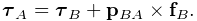If we again assume thatandare expressed in framesand, we can show that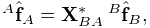(A.28)

where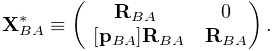(A.29)